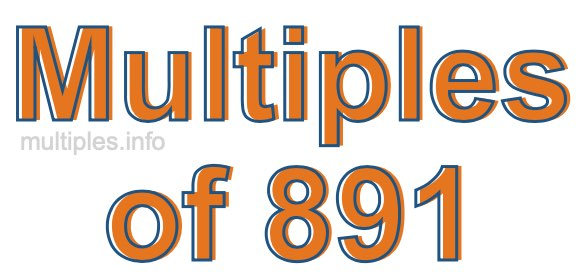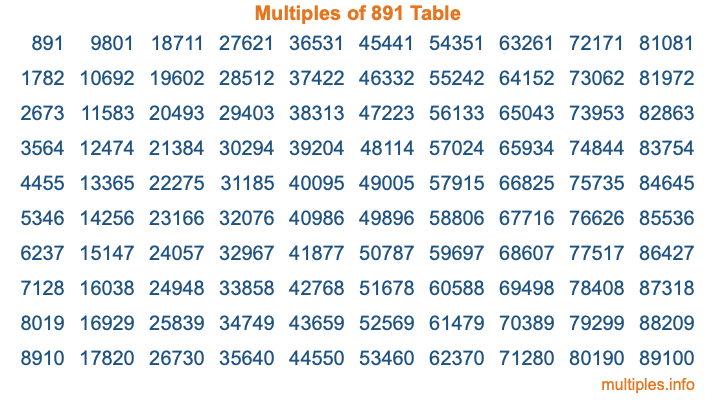Multiples of 891Welcome to the Multiples of 891 page. Here we will first teach you everything you will ever need to know about the multiples of 891, and then give you a study guide summary of everything we taught you to make sure you remember it all. Use this page to look up facts and learn information about the multiples of 891. This page will make you a multiples of eight hundred ninety-one expert!

Definition of Multiples of 891
Multiples of 891 are all the numbers that when divided by 891 equal an integer. Each of the multiples of 891 are called a multiple. A multiple of 891 is created by multiplying 891 by an integer.

Therefore, to create a list of multiples of 891, you start with 1 multiplied by 891, then 2 multiplied by 891, then 3 multiplied by 891, and so on for as long as you want. Thus, the list of the first five multiples of 891 is 891, 1782, 2673, 3564, and 4455. To see a larger list of multiples of 891, see the printable image of Multiples of 891 further down on this page. We also have a category where you can choose any nth multiple of 891.

Multiples of 891 Checker
The Multiples of 891 Checker below checks to see if any number of your choice is a multiple of 891. In other words, it checks to see if there is any number (integer) that when multiplied by 891 will equal your number. To do that, we divide your number by 891. If the the quotient is an integer, then your number is a multiple of 891.

Is  a multiple of 891?

Least Common Multiple of 891 and ...
A Least Common Multiple (LCM) is the lowest multiple that two or more numbers have in common. This is also called the smallest common multiple or lowest common multiple and is useful to know when you are adding our subtracting fractions. Enter one or more numbers below (891 is already entered) to find the LCM.

Check out our LCM Calculator if you need more details about the Least Common Multiple or if you need the LCM for different numbers for adding and subtraction fractions.

nth Multiple of 891
As we stated above, 891 is the first multiple of 891, 1782 is the second multiple of 891, 2673 is the third multiple of 891, and so on. Enter a number below to find the nth multiple of 891.

th multiple of 891

Multiples of 891 vs Factors of 891
891 is a multiple of 891 and a factor of 891, but that is where the similarities end. All postive multiples of 891 are 891 or greater than 891. All positive factors of 891 are 891 or less than 891.

Below is the beginning list of multiples of 891 and the factors of 891 so you can compare:

Multiples of 891: 891, 1782, 2673, 3564, 4455, etc.

Factors of 891: 1, 3, 9, 11, 27, 33, 81, 99, 297, 891

As you can see, the multiples of 891 are all the numbers that you can divide by 891 to get a whole number. The factors of 891, on the other hand, are all the whole numbers that you can multiply by another whole number to get 891.

It's also interesting to note that if a number (x) is a factor of 891, then 891 will also be a multiple of that number (x).

Multiples of 891 vs Divisors of 891
The divisors of 891 are all the integers that 891 can be divided by evenly. Below is a list of the divisors of 891.

Divisors of 891: 1, 3, 9, 11, 27, 33, 81, 99, 297, 891

The interesting thing to note here is that if you take any multiple of 891 and divide it by a divisor of 891, you will see that the quotient is an integer.

Multiples of 891 Table
Below is an image of the first 100 multiples of 891 in a table. The table is in chronological order, column by column. The first column has the first ten multiples of 891, the second column has the next ten multiples of 891, and so on.The Multiples of 891 Table is also referred to as the 891 Times Table or Times Table of 891. You are welcome to print out our table for your studies.

Negative Multiples of 891
Although not often discussed or needed in math, it is worth mentioning that you can make a list of negative multiples of 891 by multiplying 891 by -1, then by -2, then by -3, and so on, to get the following list of negative multiples of 891:

-891, -1782, -2673, -3564, -4455, etc.

Multiples of 891 Summary
Below is a summary of important Multiples of 891 facts that we have discussed on this page. To retain the knowledge on this page, we recommend that you read through the summary and explain to yourself or a study partner why they hold true.

There are an infinite number of multiples of 891.

A multiple of 891 divided by 891 will equal a whole number.

891 divided by a factor of 891 equals a divisor of 891.

The nth multiple of 891 is n times 891.

The largest factor of 891 is equal to the first positive multiple of 891.

891 is a multiple of every factor of 891.

891 is a multiple of 891.

A multiple of 891 divided by a divisor of 891 equals an integer.

891 divided by a divisor of 891 equals a factor of 891.

Any integer times 891 will equal a multiple of 891.

Multiples of a Number
Here you can get the multiples of another number, all with the same attention to detail as we did for multiples of 891 on this page.

Multiples of
Multiples of 892
Did you find our page about multiples of eight hundred ninety-one educational? Do you want more knowledge? Check out the multiples of the next number on our list!